怎么把一个文件夹下边的文件都压缩成zip包

年薪达不到25W大数据工程师、拿不到Offer全额退款->>>以下是问题补充：

@大大g：忘记说了　压缩包的文件名要和原文件相同 (2013/11/11 12:49)

1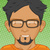winrar.exe a -k -s -ibck d:\bak.zip d:\zip\

0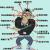[a-z0-9]{32} 指的是32位长的小写字母或数值，可以根据需要改动。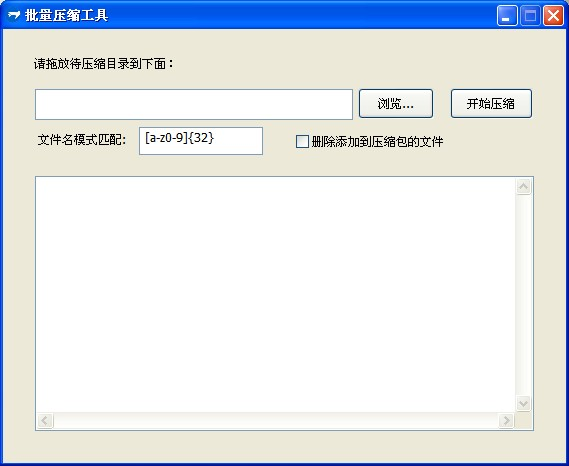import win.ui;
/*DSG{{*/
var winform = ..win.form( text="批量压缩工具";acceptfiles=1;bottom=433;max=false;right=562;border="dialog frame" )
lbParttern={ bottom=126;text="文件名模式匹配:";left=35;top=103;transparent=1;z=6;right=127;cls="static" };
txtPath={ bottom=91;right=350;left=32;top=60;z=1;edge=1;cls="edit" };
chkDelete={ bottom=121;text="删除添加到压缩包的文件";left=293;top=104;z=8;right=473;cls="checkbox" };
txtLog={ bottom=402;right=531;left=32;multiline=1;top=147;z=4;vscroll=1;hscroll=1;edge=1;cls="edit" };
button={ bottom=90;color=0;text="开始压缩";left=447;top=59;z=5;right=530;cls="button" };
static={ bottom=44;right=209;left=31;top=27;transparent=1;z=2;text="请拖放待压缩目录到下面：";cls="static" };
txtParttern={ text="[a-z0-9]{32}";bottom=126;right=260;left=136;multiline=1;top=98;z=7;edge=1;cls="edit" };
btnDir={ bottom=90;text="浏览...";left=355;top=59;z=3;right=431;cls="button" }
)
/*}}*/

import fsys;
import zlib.zip;
zipDir = function(dir,findName,delFile){

var files = {};
fsys.enum( dir, "*.*",
function(dir,filename,fullpath,findData){
if(filename && ..string.match(filename,findName)){
..table.push(files,fullpath);
}
},false
);

var zipName = fsys.path.removeBackslash(dir) + ".zip"
var zip = zlib.zip( zipName,dir );
for(k,file in files ){
for len in zip.eachWriteFile(file, , , ){
}

if(delFile){ fsys.delete(file)}
}
zip.close()
}

import fsys;
winform.button.oncommand = function(id,event){
var path = io.fullpath(winform.txtPath.text);
if(!#path){
winform.msgboxErr("请指定正确目录","批量压缩工具");
return;
}

var findName = winform.txtParttern.text;
var delFile = winform.chkDelete.checked;
zipDir(path,findName,delFile);
fsys.enum( path, "*.*",
function(dir,filename,fullpath,findData){
},
function(dir){
winform.txtLog.appendText(dir,'\r\n');
zipDir(dir,findName,delFile);
return true;
}
);

}

import fsys.dlg;
winform.btnDir.oncommand = function(id,event){
winform.txtPath.text = fsys.dlg.opendir(,winform.hwnd,"请选择待处理目录");
}

winform.wndproc = function(hwnd,message,wParam,lParam){
select(message) {
case 0x233/*_WM_DROPFILES*/ {
winform.txtPath.text = win.getDropFile(wParam );
}
}
}

winform.show()
win.loopMessage();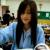0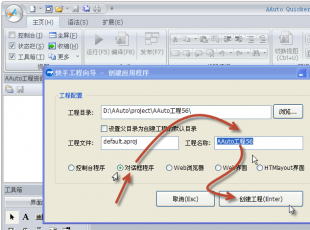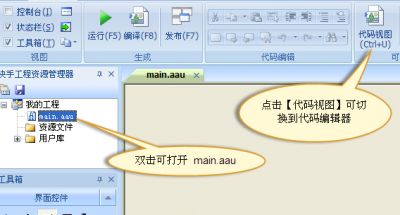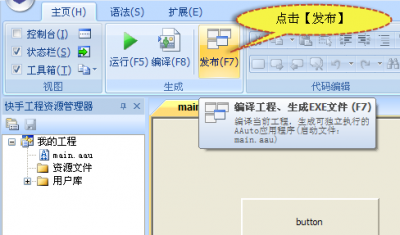0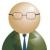0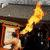引用来自“huan”的答案

0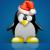0

dir temp /b /s >filst.txt